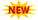WBSSC Krishi Prayukti Sahayak Recruitment || TSSPDCL AE Notification || PPSC Naib Tehsildar Admit Card ||

# NCERT Solutions For Class 1 Maths – Math Magic Solutions

NCERT Solutions For Class 1 Maths all chapters are covered in on this page. These NCERT Solutions are solved by experts in the subject with good experience in teaching it to the students. We have constructed this NCERT Solutions according to latest CBSE revised syllabus. Students can rely on this material for better understanding of concepts and thorough knowledge. Practicing question and answers in this Class 1 Maths NCERT Solutions enables students good marks in exams also.

## NCERT Solutions Class 1 Maths – CBSE Class 1 Maths Magic

All of the NCERT Solutions Class 1 Maths are answered in this article. Solutions are arranged in separate posts. Direct links to the chapter wise NCERT Solutions Maths Class 1 are provided in the following section. Students can browse to this page by just clicking on the chapter names listed below.

## NCERT Solutions For Class 1 Maths – All Chapters

• Chapter 1 Shapes and Space
• Chapter 2 Numbers from One to Nine
• Chapter 4 Subtraction
• Chapter 5 Numbers from Ten to Twenty
• Chapter 6 Time
• Chapter 7 Measurement
• Chapter 8 Numbers from Twenty-one to Fifty
• 9 Data Handling
• Chapter 10 Patterns
• Chapter 11 Numbers
• Chapter 12 Money
• Chapter 13 How Many

### NCERT Solutions Class 1st Maths Magic

Class 1 NCERT Solutions for Maths deals with the basic things about mathematics and calculating stuff, recognizing shapes, addition, subtraction, counting numbers, measuring things, etc. Learning these things are very important to for the higher studies. There is nothing big will happen if you fail a simple test in class 1. But it is very important to learn and understand these basics as these are very necessary in NCERT Solutions Class 1 Maths.